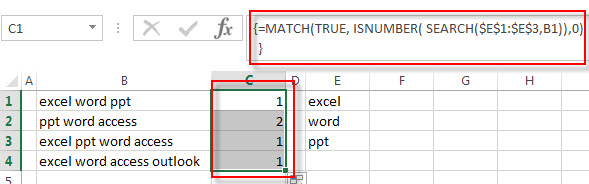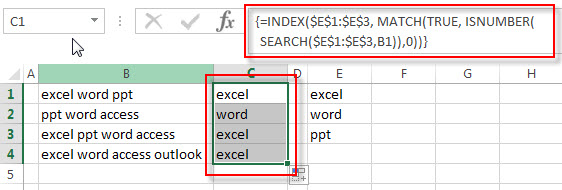# How to get first match that cell contains one of several values in a range

This post explains that how to get the first match that cell contains one of several values from another range or a list in excel. before we talked the way that how to check a cell to see that if it contains one of several values in a range or list using the SEARCH function with ISNUMBER function. So now we still need to get the first match that found in the range or list.

## Get First Match that Cell Contains one of several values

Assuming that you have a list of text strings in the range B1:B3 and you want to check each text string if it contains one of several values from the range E1:E3. If it contains any of text string in range E1:E3, then it should return the first match value found in the range E1:E3, otherwise, it should return the #N/A error. you can use a combination of the INDEX function, the MATCH function, the ISNUMBER function and the SEARCH function to create a new excel array formula as follows:

`=INDEX(\$E\$1:\$E\$3, MATCH(TRUE, ISNUMBER( SEARCH(\$E\$1:\$E\$3,B1)),0))`

Let’s see how this formula works:

=SEARCH(\$E\$1:\$E\$3,B1)

The SEACH function returns position of the first character of find_text in a text string. And this formula will search each value in the range E1:E3 inside within_text in Cell B1, if it found the match, then returns position of each text string in Cell B1, otherwise, it will return the #VALUE! Error. So it will return an array result like this:

`{1;7;12}`

The returned result goes into the ISNUMBER function as its argument.

=ISNUMBER(SEARCH(\$E\$1:\$E\$3,B1))

The ISNUMBER function will check if a cell contains a numeric value, and this formula will check each items of array result returned by the SEACH function, if the item is a numeric value, then return TRUE, otherwise, returns FALSE. So it will return another array result like this:

`{TRUE;TRUE;TRUE}`

=MATCH(TRUE, ISNUMBER( SEARCH(\$E\$1:\$E\$3,B1)),0)The MATCH function will search the first value that is exactly equal to the lookup_value “TRUE”, then return the position of the first match. So this formula returns 1. It goes into the INDEX function as its row_num argument.

=INDEX(\$E\$1:\$E\$3, MATCH(TRUE, ISNUMBER( SEARCH(\$E\$1:\$E\$3,B1)),0))The index function will extract a value from the range E1:E3 based on the position number returned by the MATCH function. So it should be returned the first match value as “excel”.

### Related Formulas

• Check If Cell Contains All Values from Range
If you want to check if a cell contains all values in a list, you can use a combination of the SEARCH function, the SUMPRODUCT function, the ISNUMBER function and COUNTA function…
• Get last match that cell contains one of several values in a range
If you want to check a cell that if it contains one of several values in a range, you can use the SEARCH function to search find_text in a range inside a text string. Then we can use the LOOKUP function to get the last match values….
• Check if Cell contains one of many values from range
Assuming that you have a list of text strings in the range B1:B3 and you want to check each text string if it contains one of several values in a range E1:E3. If it contains any of text string in range E1:E3, then it should be return TRUE value, otherwise, it should return FALSE….

### Related Functions

• Excel INDEX function
The Excel INDEX function returns a value from a table based on the index (row number and column number)The INDEX function is a build-in function in Microsoft Excel and it is categorized as a Lookup and Reference Function.The syntax of the INDEX function is as below:= INDEX (array, row_num,[column_num])…
• Excel SEARCH function
The Excel SEARCH function returns the number of the starting location of a substring in a text string.The syntax of the SEARCH function is as below:= SEARCH  (find_text, within_text,[start_num])…
• Excel MATCH function
The Excel MATCH function search a value in an array and returns the position of that item.The syntax of the MATCH function is as below:= MATCH  (lookup_value, lookup_array, [match_type])….
• Excel ISNUMBER function
The Excel ISNUMBER function returns TRUE if the value in a cell is a numeric value, otherwise it will return FALSE.The syntax of the ISNUMBER function is as below:= ISNUMBER (value)…